### BUILDING A CROSSOVER FILTER

Three major components are used to build a Crossover Filter: Resistors, Capacitors and Inductors. All are passive components connecting in series or in parallel to each other and to the Loudspeaker Driver.

-So what is the idea behind a set of passive components forming a Crossover Filter ?

A filter needs to establish a Gain ratio between its output and input voltage. This Gain is expected to be frequency dependent. In this way the filter will manage to provide attenuation and shape control of the Loudspeaker's SPL Response. Frequencies for which this ratio is less than unity (or 0 dB) will be attenuated.

The notion hidden behind the complex mathematics of the Gain Response of a Crossover Filter, is the Voltage Divider.

 Figure on the right describes how voltage is distributed (divided) around a series of components. Output voltage is selected by one of these components. The Gain of this circuit is simply determined by the ratio of component resistances. Let us have an example : Let R1 be 9 Ohms and R2 be 1 Ohm. Input voltage Vi is applied on the series of R1 and R2. Output Voltage Vo is pulled out of R2. This Voltage Divider makes Vo equal to 1/(9+1) = 1/10. In dB's this ratio becomes 20 * log (0.1)=-20dB Apparently Vo is only a small fraction of Vi. Generally speaking in a Voltage Divider, low resistances at the output furnish small fractions of the applied (input) voltage. If we reverse resistance values (R1=1 Ohm, R2=9 Ohms) this will be easily confirmed: Now the Gain becomes 9/(9+1)=0.9 ie. -1dB ! Output voltage is only 1dB less than the applied (input) voltage. So high values of resistance at the output furnishes large fraction of the applied signal.-What all these numerical divisions have to do with Crossover Filters ?

Well it is quite ..simple: If we drop using (fixed value) resistors and manage to get components that change their 'resistance' with frequency, we will end up with a voltage divider that changes with frequency; so will change its Gain. In other words we will end up with a frequency dependent Gain. This is the case of a filter.

-Which components have variable resistance?

Capacitors and Inductors exhibit a 'resistance' strongly dependent to frequency.

-Could we elaborate on this ?

When dealing with circuits in which AC signals flow (like music), we prefer using Impedance Z instead of resistance. Impedance is also measured in Ohms. It also abides to Ohm's Law for voltages (V) and currents (I) : I=V/Z

The Impedance of a plain 4.7 Ohm resistor is simply 4.7 Ohm. A Capacitor has a large value of Impedance at low frequencies. Its Impedance drops quickly with increasing frequency. Inductors are completely different. Their Impedance is very low at bass frequencies, getting higher with increasing frequency. Let us have a look on this.

For simplicity we use f for frequency though in most equations of our analysis we prefer using angular frequency ω which is proportional to frequency : ω = 2 π f.

Impedance is an arithmetic quantity that belongs to the field of Complex Numbers. In general it has a real and an imaginary part; both measured in Ohms. To use Ohm's Law we take magnitudes of all complex quantities.

A plain resistor R has no imaginary part in its Impedance : Z(R) = R + 0*i, where i is the famous imaginary unit (square root of -1). Any component in Electronics with Impedance having a zero imaginary part is said to be resistive.

A capacitor C ideally has no real part in its Impedance : Z(C) = 0 - i/Cω, its imaginary part is negative and decreases with frequency. Negative sign only relates voltage and current oscillations; it does not interfere with the main idea of Ohm's Law.

Thus if a capacitor has an Impedance of -12.5i Ohms (at a specific frequency) and we supply a signal of 2.5Vrms across its terminals, a current of 2.5/12.5=0.2 Amps rms will flow through. The division operation required in Ohm's Law only takes magnitudes of complex numbers involved. No need to worry about i.

Any component in nature with Impedance having a negative imaginary part is said to be capacitive.

An inductor L ideally has no real part in its Impedance too : Z(L) = 0 + Lωi, its imaginary part is positive and increases with frequency. Thus if an inductor has an Impedance of 1.8i Ohms (at a specific frequency) and we supply a signal of 540mVrms across its terminals, a current of 540/1.8=300mA rms will flow through. Any component with Impedance having a positive imaginary part is said to be inductive.

-What about real-world capacitors and inductors ?

Electronics is a vast part of Science. Specialized research has suggested complicated models for capacitors' and inductors' impedance. Electrolytic capacitors can not follow the -i/Cω model down to very-very low frequencies.This formula gives huge Ohm values (MΩ or more) that only an insulating material would match. Unfortunately their construction features a 'leak' resistance of several kΩ that bypasses this (theoretically infinite at 0Hz) insulating value. MKT, MKP or MKC capacitors give an excellent performance in this area.

Real inductors follow a simpler impedance model. The real part of their impedance is not zero. It is given by the resistance of their copper wire. For a typical L=1mH inductor with a moderate 1mm wire diameter this resistance is no more than a fraction of an Ohm. This resistance is usually proportional to the wire length used for the inductor's winding.

For a good degree of accuracy in Crossover Design we can use a fixed value of 0.1 Ohms for most inductors:

Z(L) = 0.1 + Lωi.

-Could we have a worked example of a LowPass Filter with real-world components ?

We will present a simple LowPass Filter based on the 2-component Voltage Divider illustrated at the beginning of this article. We will use an Inductor L=1mH in place of R1 and a simplified model of an 8 Ohm Loudspeaker in place of R2.

 The idea behind the operation of this simplified filter is simple: At low frequencies the Inductor has a low impedance (compared to the 8 Ohm Loudspeaker) so the voltage division scheme gives an output voltage very close to input. Gain ratio is almost unity or 0dB. At higher frequencies the impedance of the Inductor becomes very large; Output voltage is now only a small fraction of input voltage. Gain drops with frequency. Filter attenuation has been accomplished.Let us use numbers and some arithmetic.

At 100Hz our inductor has an imaginary impedance of Lωi=0.001*2*π*100*i=0.63*i Ohms only.

At 10000Hz the impedance of the same inductor becomes 0.001*2*π*10000*i= 63*i Ohms !

Gain at 100 Hz equals 8 / |8+0.63i| = 8 / √(8^2+0.63^2) = 8 / 8.025 = 0.997 = 1 approximately or 0dB.

For Gain calculations we use | .. | to take the magnitude of any complex impedance expression. For example the magnitude of 3+4i Ohms is |3+4i| = √(3^2+4^2) = √(9+16) = √25 = 5 Ohms.

Gain at 10 kHz equals 8 / |8+63i| = 8 / √(8^2+63^2) = 8 / 63.5 = 0.126 or -18dB !

A plot of the Gain Response of this circuit confirms that it acts as a LowPass Filter. Don't hesitate to check our calculations with the actual graph.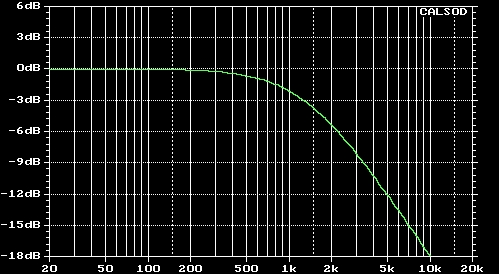-Could we do the same with a HighPass Filter ?

We will use a Capacitor C=6.8uF in place of R1 and a simplified model of an 8 Ohm Loudspeaker in place of R2.

 The idea behind the operation of this filter is also simple: At low frequencies the Capacitor has a huge impedance (compared to the 8 Ohm Loudspeaker) so the voltage division scheme gives an output voltage very close to zero. Gain ratio in dB is highly negative. At higher frequencies the impedance of the Capacitor drops to almost zero; Output voltage is now almost equal to input voltage. Gain becomes approximately unity. This Filter attenuates only low frequencies.Let us do some arithmetic.

At 100Hz our Capacitor has an imaginary impedance of -i/Cω=-i / (6.8*10-6*2*π*100)= -234*i Ohms.

At 10000Hz the impedance of the same Capacitor becomes -2.34*i Ohms !

Gain at 100 Hz equals 8 / |8-234i| = 8 / √(8^2+234^2) = 8 / 234.1 = 0.034 or -29.3dB !

Gain at 10 kHz equals 8 / |8-2.34i| = 8 / √(8^2+2.34^2) = 8 / 8.335 = 0.96 or 0dB approx.

A plot of the Gain Response of this circuit confirms that it acts as a HighPass Filter.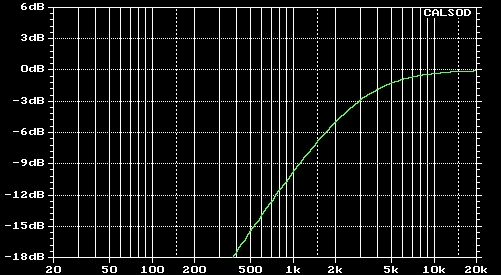-What if we want a more "steep" Gain Response?

Well there is a connection between the roll-off rate (dB per octave) and the components connected to the Loudspeaker Driver. In LowPass Filters one inductor usually creates Gain Responses of the First Order ie. with a roll-off rate of -6dB per octave. Respectively one capacitor (in a HighPass Filter) will also give a First Order Gain Response. Such roll-off rates are inadequate for most HiFi Speaker Systems. Second Order Filters (or even Third and Fourth Order) are much more popular.

To make a Second Order Filter with a 12dB/octave roll-off rate we must use an additional component next to the filter's load (ie. the Loudspeaker Driver) in order to decrease its Impedance at frequencies where attenuation is desired.

Let's take the example of the LowPass Filter above. Attenuation is desired at higher frequencies. At 10kHz the Loudspeaker Driver has an 8 Ohm fixed impedance while the inductor has risen to a 63i Ohms value. Voltage Divider is based on Impedance ratio giving a Gain Response of 8 / |8+63i| = 8 / √(8^2+63^2) = 8 / 63.5 = 0.126 or -18dB as already stated. If we place in parallel to the Driver a capacitor (for example a 10uF) having a small Impedance at 10kHz, this parallel combination will give an impedance value much less than 8Ohms. Thus our Voltage Divider will give a smaller Gain value ie. a higher attenuation.

So to make a Second Order LowPass Filter we use a capacitor in parallel to the Loudspeaker Driver. This action short-circuits the Loudspeaker Driver at higher frequencies producing more negative dB values of Gain Response.

In a similar manner we place an inductor in parallel to the Loudspeaker Driver for HighPass Filters of Second Order. This action short-circuits the Loudspeaker Driver at lower frequencies producing more negative dB values of Gain attenuation.

Let us elaborate on this:

 Figure on the right  illustrates a Second Order LowPass Filter having a capacitor in parallel to the Loudspeaker Driver. If we extend our previous design example (L=1mH) with the addition of a 10uF capacitor we get the following results at 10 kHz : The capacitor's impedance is -i/(0.00001*2*π*10000) =-1.59i Ohms. This value in parallel to the ideal 8 Ohm Loudspeaker Driver load produces a total of (8 // -1.59i) Ohms for this combimation. Symbol '//' stands for the parallel combination of impedances. Its computation involves complex arithmetic operations. In general this combination is evaluated as the ratio of the product of these impedances to their sum.In our case this combination gives (0.3-1.53i) Ohms. Obviously the capacitor has managed to short-circuit the 8 Ohm value of the driver's impedance down to a value with a much smaller magnitude √(0.3^2+1.53^2) = 1.56 Ohms !

If we substitute the above values in the expression shown on this figure and get magnitude values for the complex Gain Vo/Vi, we end up with a -32 dB value !

We notice that input voltage is no longer distributed across the 63i Ohms of the inductor and the 8 Ohms of the Loudspeaker Driver. The capacitor decreases the latter down to an absolute value of 1.56 Ohms. Inductor's absolute impedance of 63 Ohms is dominant in the Voltage Divider scheme and output voltage is only a small fraction of input. Attenuation has been increased efficiently.

 Figure on the right illustrates a Second Order HighPass Filter having a capacitor in parallel to the Loudspeaker Driver. If we extend our corresponding design example (C=6.8uF) with the addition of a 0.7mH inductor we get the following results at 100 Hz: Capacitor has a -234i Ohms impedance. Inductor gives a small value of 0.44i Ohms. The latter is in parallel to the 8 Ohms of the ideal Loudspeaker Driver. This parallel combination gives a very low value of impedance (with a magnitude of less than 0.5 Ohms). Obviously the capacitor impedance dominates and thence output voltage is practically zero. High attenuation has been accomplished at 100 Hz.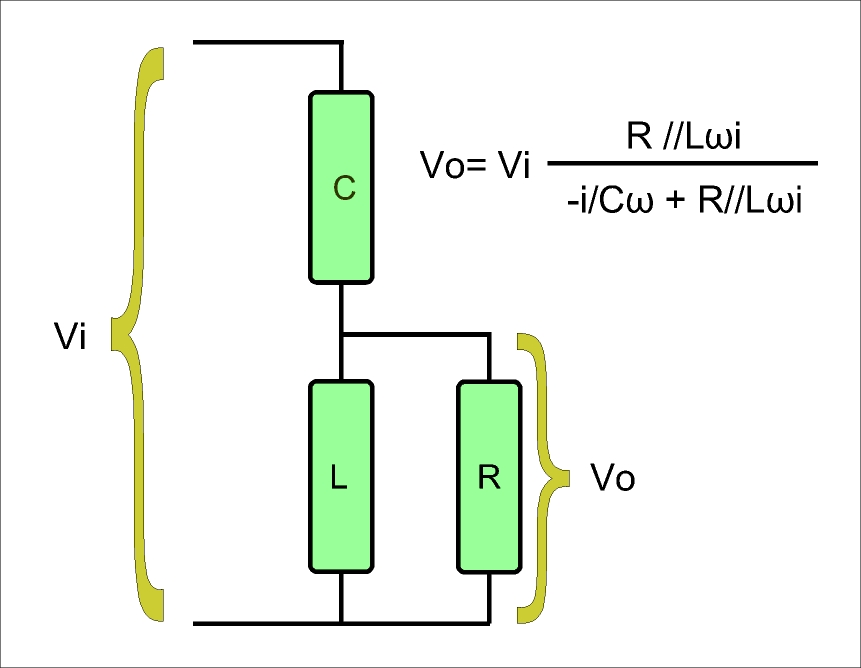Gain Responses of our example 2nd Order LowPass and HighPass Filters.

-Should we evaluate all this complex operations for all the components of a Crossover Network and for several frequency values?

Apparently not! We use Circuit Simulation Software where we describe the filters' structure in terms of component nodes and values. We then define a frequency range for our analysis (100 Hz to 20000Hz for example) and tell the software to produce the necessary Gain Responses.

-Who decides these filters' structure?

Well there are some criteria for the selection of the filters' order. We better let this issue be discussed in design examples later on.

-Who decides the filters' component values?

Well there are several formulas evaluating their filters' cutoff frequencies as a function of component values. Yet these formulas assume that purely resistive loads (ie the Loudspeaker Drivers) are connected to them. This is unfortunately not the case. Hence we end up with no useful formulas for Crossover Network Design..

A designer can only use a simulation software repetitively to get optimum component values.

-What do we mean when we assert that an 8 Ohm Loudspeaker Driver does not have an (ideal) resistive impedance?

The following figures show a plot of the magnitude of the complex impedance of a Woofer and a Tweeter Driver for all audible frequencies. No comments.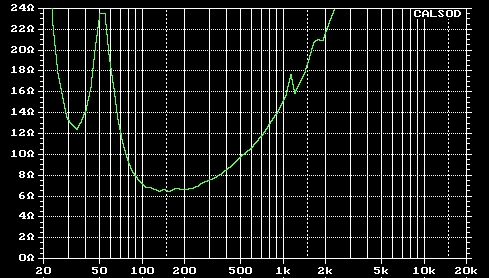(Magnitude of complex) impedance of a typical 10 inch Woofer Driver.

Apparently this impedance is highly frequency dependent. The rise above 300Hz is due to the Voice Coil inductance. The double resonance below 100Hz is due to the enclosure air coupling with the driver's cone mass and suspension.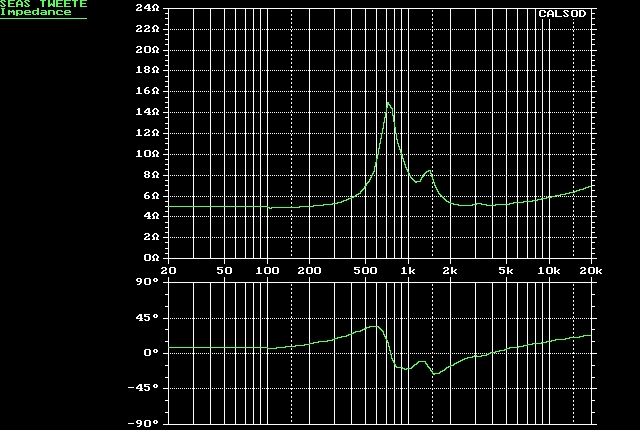(Magnitude and Phase of complex) impedance of a typical 1 inch Tweeter Driver.

For Tweeters impedance is less frequency dependent. The rise above 5000Hz is due to the Voice Coil inductance. The double resonance at 700 and 1400Hz is due to the chamber's air stiffness coupling with the dome mass and its suspension.

The effect of these non-ideal and non-fixed impedances on the Gain Responses of our 1st Order filter examples is illustrated in the following figure :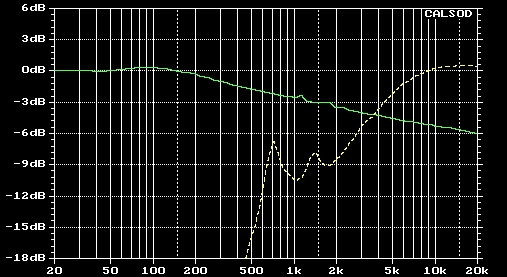Our LowPass Filter has failed to achieve even its theoretical 6dB/octave roll-off rate. The HighPass Filter has deformed completely. Component values must be reconsidered if these filters are to be connected to real-world drivers.

The effect of the same non-ideal driver impedances on the Gain Responses of our 2nd Order filter examples is investigated in the following figure :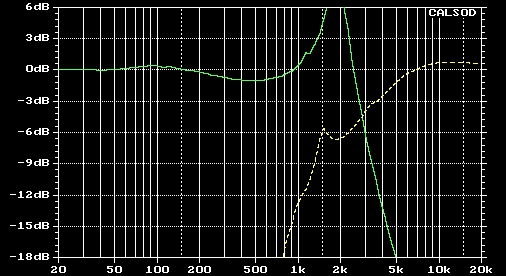Our LowPass Filter exhibits a strong resonance while the HighPass Filter has deformed partially. Component values must also be reconsidered if these filters are to be connected to real-world drivers.

-So how do we deal with this serious problem?

We measure and store values of our Loudspeaker Drivers' impedances over a wide frequency range (usually 10Hz to 40khz).

We decide filter structure and start-up component values. We place all these along with driver impedance values' set into our simulation software and ..push the button. We repeatedly change component values until a good shape of Gain Responses is achieved. If we can't get it done we change filter structure by changing filters' order. Several hours of continuous effort may be necessary for that. Be prepared !